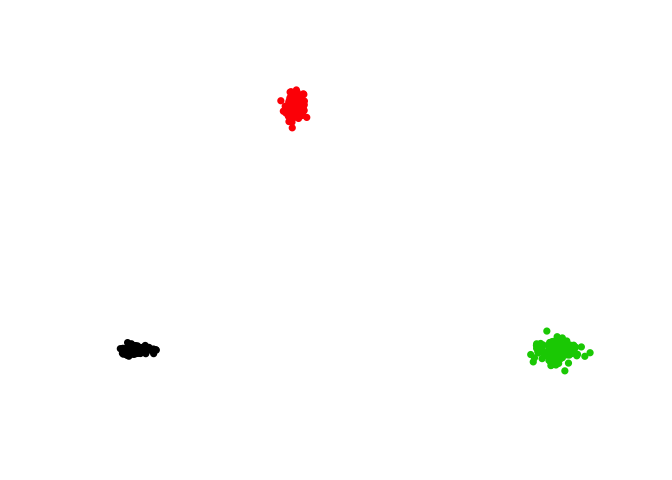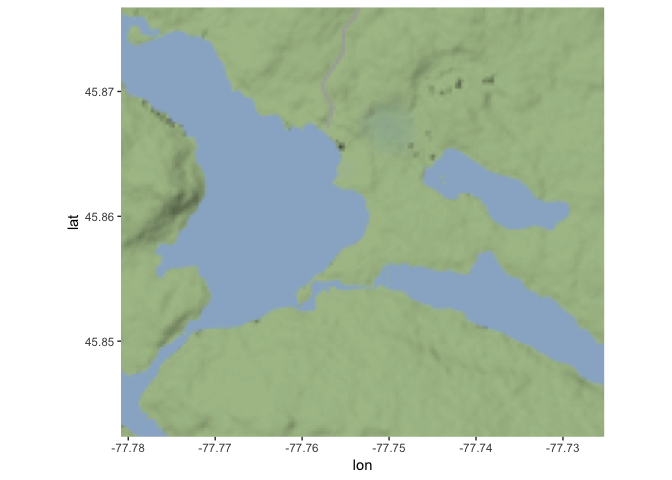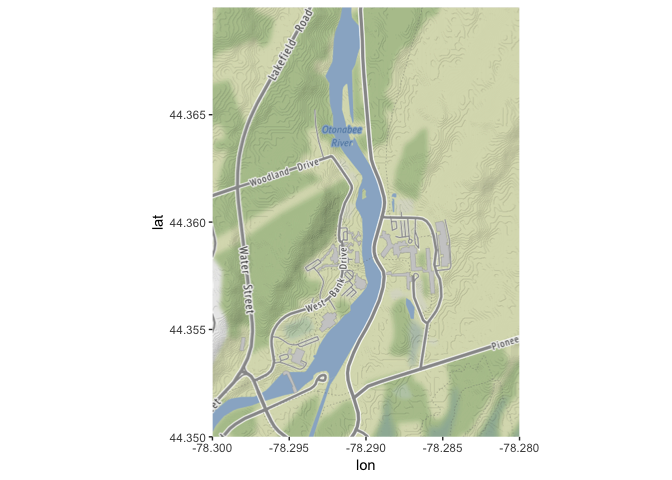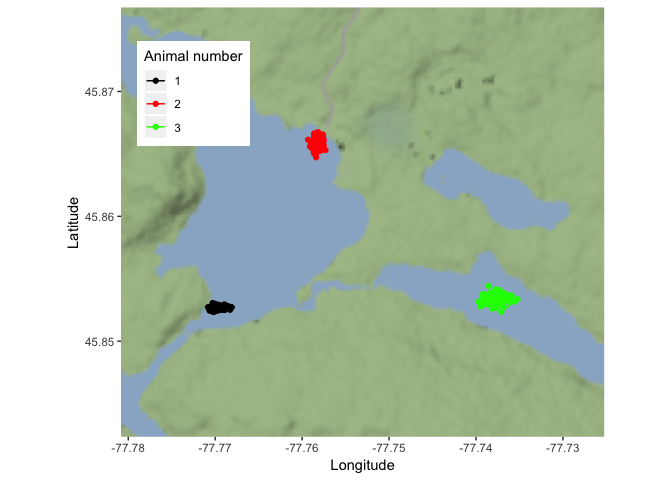## Introduction

This is the first of several posts based on a workshop I developed as an introduction to analyzing telemetry data in R. I will use a simulated data set to demonstrate basic movement analyses in R for telemetry data.

Update 2020-04-02 I have updated the ggmap section to account for Google’s change in API. The tutorial now uses Stamen tiles, but I included code for downloading Google tiles and a link on how to register for a key.

Outline

1. Simulate data and format for spatial analyses using the sp package
2. Create a map of telemetry points (and paths) using the ggmap package

## 1. Load data and format for spatial analyses

Let’s simulate some simple data for three animals as an example.

# Create potential telemetry data points for 3 animals with 100 relocations each.
points.1.df <- data.frame(id = 1,  # identifies the animal
x = rnorm(100, mean = 284979, sd = 45),  # easting coordinates
y = rnorm(100, mean = 5081411, sd = 15), # northing coordinates
zone = 18, # UTM zone
date = seq(as.Date("2017/05/03"), as.Date("2017/08/10"), by = 1)) # sequence of dates

points.2.df <- data.frame(id = 2,
x = rnorm(100, mean = 285910, sd = 30),
y = rnorm(100, mean = 5082848, sd = 50),
zone = 18,
date = seq(as.Date("2017/05/03"), as.Date("2017/08/10"), by = 1))

points.3.df <- data.frame(id = 3,
x = rnorm(100, mean = 287448, sd = 70),
y = rnorm(100, mean = 5081395, sd = 45),
zone = 18,
date = seq(as.Date("2017/05/03"), as.Date("2017/08/10"), by = 1))

points.df <- rbind(points.1.df, points.2.df, points.3.df) # join the three animals' data together

# To format for spatial analyses, we need to remove NA values from the coordinate columns
# This isn't an issue for this simulated dataset
points.df <- points.df[!is.na(points.df$x) & !is.na(points.df$y),]

# The dataframe should only have 3 columns (x, y, and an identifier)
# for calculating trajectories and home ranges.
points.sp <- points.df[, c("id", "x", "y")]

# Turn into a spatial points dataframe (class: SpatialPointsDataFrame)
library(sp)
coordinates(points.sp) <- c("x", "y")

# Examine the structure of our SpatialPointsDataFrame
str(points.sp)
## Formal class 'SpatialPointsDataFrame' [package "sp"] with 5 slots
##   ..@ data       :'data.frame':  300 obs. of  1 variable:
##   .. ..$id: num [1:300] 1 1 1 1 1 1 1 1 1 1 ... ## ..@ coords.nrs : int [1:2] 2 3 ## ..@ coords : num [1:300, 1:2] 284973 284962 285019 284983 284942 ... ## .. ..- attr(*, "dimnames")=List of 2 ## .. .. ..$ : chr [1:300] "1" "2" "3" "4" ...
##   .. .. ..$: chr [1:2] "x" "y" ## ..@ bbox : num [1:2, 1:2] 284882 5081287 287648 5082941 ## .. ..- attr(*, "dimnames")=List of 2 ## .. .. ..$ : chr [1:2] "x" "y"
##   .. .. ..$: chr [1:2] "min" "max" ## ..@ proj4string:Formal class 'CRS' [package "sp"] with 1 slot ## .. .. ..@ projargs: chr NA Notice the slot for “proj4string” of class ‘CRS’. This refers to the coordinate reference system data and describes how the x and y coordinates are mapped on the globe. Our example data was collected in UTM in zone 18 in the WGS84 datum. Let’s set the CRS using the proj4string function. # Set coordinate system & projection proj4string(points.sp) <- CRS( "+proj=utm +zone=18 +datum=WGS84 +units=m +no_defs" ) To learn more about coordinate reference systems and how to set them for your own data, check out: https://www.nceas.ucsb.edu/~frazier/RSpatialGuides/OverviewCoordinateReferenceSystems.pdf We can check everything is working by visualizing our data. plot(points.sp, col = points.sp@data$id, pch = 16)Everything is looking good, although we just see the points for now. Now let’s try plotting the points overtop some map features.

## 2. Mapping using ggmap

The ggmap package is a nice way to plot points, polygons, and lines on top of Google’s (and other sources’) map tiles (eg. satellite or terrain). It follows the same language as ggplot (“gg” stands for “Grammar of Graphics”). This is one way to approach to mapping spatial data in R, but there are many other options, such as Rgooglemaps and maptools.

Lets create some maps with our spatial points.

Before we get started on making a map, we need to convert our SpatialPointsDataFrames into latitude and longitude for ggmap using the spTransform function.

# Transform the point object (points.sp)
points.sp.geo <- spTransform(points.sp, CRS("+proj=longlat +ellps=WGS84 +datum=WGS84 +no_defs "))

The next step to creating our map is to download the tiles for the study area using the get_map (for Google) or get_stamenmap (for Stamen) functions in the ggmap package. The get_map function requires:

• location,
• source,
• zoom, and
• maptype

The location can be the latitude and longitude, or a character string describing where you want your map centered. The zoom argument is an integer from 3 (continent) to 21 (building), with a default of 10 (city). To see the options of other source and maptype arguments, type ??get_map into R.

For get_stamenmap function, you need to set:

• bbox (stands for bounding box; the corners of the extent), and
• maptype (Stamen does not have satellite imagery)
# Plot study site using ggmap
library(ggmap)
# Plot study site using ggmap
mybasemap <- get_stamenmap(bbox = c(left = min(points.sp.geo@coords[,1])-0.01,
bottom = min(points.sp.geo@coords[,2])-0.01,
right = max(points.sp.geo@coords[,1])+0.01,
top = max(points.sp.geo@coords[,2])+0.01),
zoom = 12)

## For satellite imagery, use Google map tiles. However, you need to register a key.
## Guide on getting a key: https://www.r-bloggers.com/geocoding-with-ggmap-and-the-google-api/
# register_google(key = "yourkeyhere")
## The location argument can take a vector with latitude and longitude, or a character string.
# mybasemap <- get_map(location = c(lon = mean(points.sp.geo@coords[,1]) ,
#                                   lat = mean(points.sp.geo@coords[,2])),
#                      source = "google", zoom = 14, maptype = 'satellite')

Not that we’ve downloaded the tiles, let’s make a basic map using the ggmap function

ggmap(mybasemap) # What does it look like?If you’re using Google tiles (you need a key first), the location argument can take a vector with longitude and latitude, or a character string. For the get_stamenmap function, you need a bounding box (bbox).

Let’s download the tiles for Trent University campus.

# Google Map version
# trentumap <- get_map(location = "trent university, peterborough", zoom = 16, maptype = 'satellite')

# Stamen version
trentumap <- get_stamenmap(bbox = c(left = -78.29 - 0.01,
right = -78.29 + 0.01,
bottom = 44.36 - 0.01,
top = 44.36 + 0.01),
zoom = 15)
ggmap(trentumap)Let’s build on our basic map and add our point relocations for each animal (connected by a line).

# Turn the spatial data frame of points into just a dataframe for plotting in ggmap
points.geo <- data.frame(id = points.sp.geo@data\$id, # add individual identifier
points.sp.geo@coords)

# Plot imagery + points + paths
mymap.paths <-ggmap(mybasemap) +
geom_point(data = points.geo, aes(x = x, y = y, colour = as.factor(id))) +
geom_path(data = points.geo, aes(x = x, y = y, colour = as.factor(id))) +
theme(legend.position = c(0.15, 0.80)) +
labs(x = "Longitude", y = "Latitude") +
scale_colour_manual(name = "Animal number",
values = c("black", "red", "green"))

mymap.pathsLooking good! You will have to adjust the zoom level of the basemap depending on how far apart your features are.

I hope someone finds this useful! In upcoming posts, I will:

• construct home ranges in R with minimum convex polygons and kernels,
• create and analyze differences in animal trajectories.